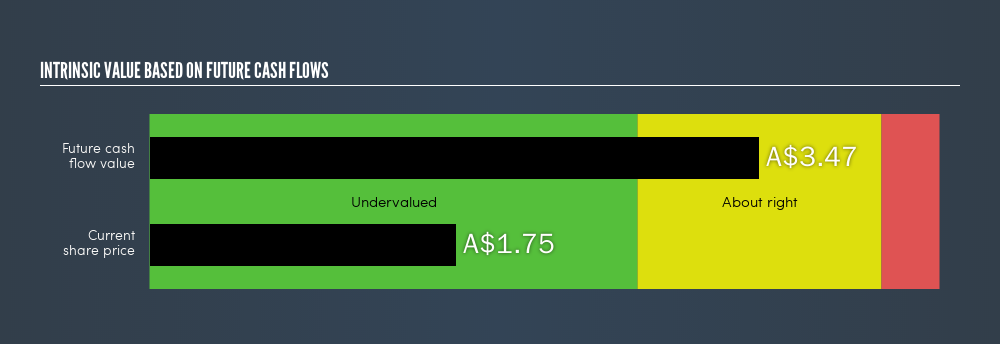latest

# Is There An Opportunity With GBST Holdings Limited’s (ASX:GBT) 49.62% Undervaluation?

Want to participate in a short research study? Help shape the future of investing tools and receive a \$20 prize!

In this article I am going to calculate the intrinsic value of GBST Holdings Limited (ASX:GBT) by taking the expected future cash flows and discounting them to their present value. I will be using the Discounted Cash Flows (DCF) model. Don’t get put off by the jargon, the math behind it is actually quite straightforward. If you want to learn more about discounted cash flow, the basis for my calcs can be read in detail in the Simply Wall St analysis model. If you are reading this and its not February 2019 then I highly recommend you check out the latest calculation for GBST Holdings by following the link below.

### The model

I use what is known as a 2-stage model, which simply means we have two different periods of varying growth rates for the company’s cash flows. Generally the first stage is higher growth, and the second stage is a more stable growth phase. To begin with we have to get estimates of the next five years of cash flows. For this I used the consensus of the analysts covering the stock, as you can see below. I then discount this to its value today and sum up the total to get the present value of these cash flows.

#### 5-year cash flow estimate

 2019 2020 2021 2022 2023 Levered FCF (A\$, Millions) A\$11.95 A\$15.70 A\$18.10 A\$13.00 A\$16.00 Source Analyst x2 Analyst x2 Analyst x2 Analyst x1 Analyst x1 Present Value Discounted @ 8.46% A\$11.02 A\$13.35 A\$14.19 A\$9.39 A\$10.66

Present Value of 5-year Cash Flow (PVCF)= AU\$59m

After calculating the present value of future cash flows in the intial 5-year period we need to calculate the Terminal Value, which accounts for all the future cash flows beyond the first stage. The Gordon Growth formula is used to calculate Terminal Value at an annual growth rate equal to the 10-year government bond rate of 2.3%. We discount this to today’s value at a cost of equity of 8.5%.

Terminal Value (TV) = FCF2023 × (1 + g) ÷ (r – g) = AU\$16m × (1 + 2.3%) ÷ (8.5% – 2.3%) = AU\$266m

Present Value of Terminal Value (PVTV) = TV / (1 + r)5 = AU\$266m ÷ ( 1 + 8.5%)5 = AU\$177m

The total value is the sum of cash flows for the next five years and the discounted terminal value, which results in the Total Equity Value, which in this case is AU\$236m. To get the intrinsic value per share, we divide this by the total number of shares outstanding, or the equivalent number if this is a depositary receipt or ADR. This results in an intrinsic value of A\$3.47. Relative to the current share price of A\$1.75, the stock is quite good value at a 50% discount to what it is available for right now.ASX:GBT Intrinsic value, February 22nd 2019

### The assumptions

The calculation above is very dependent on two assumptions. The first is the discount rate and the other is the cash flows. If you don’t agree with my result, have a go at the calculation yourself and play with the assumptions. Because we are looking at GBST Holdings as potential shareholders, the cost of equity is used as the discount rate, rather than the cost of capital (or weighed average cost of capital, WACC) which accounts for debt. In this calculation I’ve used 8.5%, which is based on a levered beta of 0.800. This is derived from the Bottom-Up Beta method based on comparable companies, with an imposed limit between 0.8 and 2.0, which is a reasonable range for a stable business.

### Next Steps:

Valuation is only one side of the coin in terms of building your investment thesis, and it shouldn’t be the only metric you look at when researching a company. What is the reason for the share price to differ from the intrinsic value? For GBT, there are three important aspects you should further examine:

1. Financial Health: Does GBT have a healthy balance sheet? Take a look at our free balance sheet analysis with six simple checks on key factors like leverage and risk.
2. Future Earnings: How does GBT’s growth rate compare to its peers and the wider market? Dig deeper into the analyst consensus number for the upcoming years by interacting with our free analyst growth expectation chart.
3. Other High Quality Alternatives: Are there other high quality stocks you could be holding instead of GBT? Explore our interactive list of high quality stocks to get an idea of what else is out there you may be missing!

PS. The Simply Wall St app conducts a discounted cash flow for every stock on the ASX every 6 hours. If you want to find the calculation for other stocks just search here.

We aim to bring you long-term focused research analysis driven by fundamental data. Note that our analysis may not factor in the latest price-sensitive company announcements or qualitative material.

If you spot an error that warrants correction, please contact the editor at editorial-team@simplywallst.com. This article by Simply Wall St is general in nature. It does not constitute a recommendation to buy or sell any stock, and does not take account of your objectives, or your financial situation. Simply Wall St has no position in the stocks mentioned. Thank you for reading.